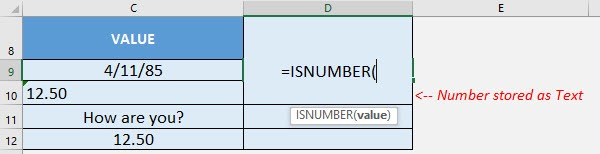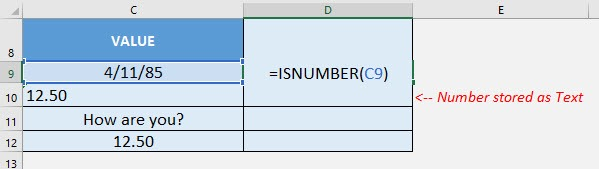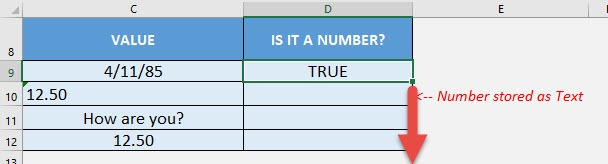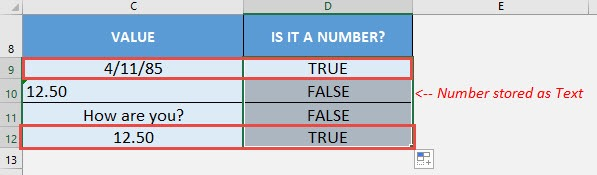What does it do?

Checks if the cell value is numeric or not

Formula breakdown:

=ISNUMBER(value)

What it means:

=ISNUMBER(value to be checked if it is a number or not)

Have a bunch of values and you need to check which ones are numbers? The ISNUMBER Formula in Excel is perfect for this!

There are a couple of interesting points to take note of the ISNUMBER Formula:

• Dates are also treated as numerical values, so the ISNUMBER Formula will return TRUE
• If the number is stored as text, then the ISNUMBER Formula will return FALSE

I explain how you can do this below:STEP 1: We need to enter the ISNUMBER function in a blank cell:

## =ISNUMBER(STEP 2: The ISNUMBER arguments:

## value

What is the value to be checked?

Select the cell containing the value you want to be checked if it is a number:

## =ISNUMBER(C9)Apply the same formula to the rest of the cells by dragging the lower right corner downwards.You can now see which ones are the numbers!How to Use the ISNUMBER Formula in Excel